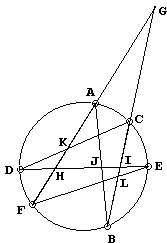## Pascal's Theorem: What is it? A Mathematical Droodle

A few wordsPascal's theorem is a direct generalization of that of Pappus. Its dual is a well known Brianchon's theorem.

The theorem states that if a hexagon is inscribed in a conic, then the three points at which the pairs of opposite sides meet, lie on a straight line. The theorem is clearly projective. If it holds for one kind of conics, it holds for any other. In particular, if it holds for a circle, it also holds for a pair of intersecting straight lines. (As a projective property, it then also holds for a pair of parallel lines.) This is why it is said to be a generalization of the theorem of Pappus. Below we consider the case when the six points lie on a circle. (Elsewhere there is an illustration of the Pascal's theorem on an ellipse and a proof based on Chasles' theorem and a direct proof in homogeneous coordinates.)

There are 60 quadrilaterals with vertices at the given six points; relative positions of the points with respect to each other also affect the appearance of the hexagon. For example, some sides may be parallel, in which case the proof below will have to be modified.Assume the configuration is as depicted on the left. We are to prove that the points J, K, L are colinear. We shall apply the theorem of Menelaus to ΔGHI and its three transversals: DKC, AJB and ELF.

Transversal Menelaus' Theorem DKC HK/GK · GC/IC · ID/HD = 1 AJB HA/GA · GB/IB · IJ/HJ = 1 ELF HF/GF · GL/IL · IE/HE = 1

Multiply the three identities and rearrange the terms:

HK/GK · GL/IL · IJ/HJ · (ID·IE/IB·IC) · (HF·HA/HD·HE) · (GC·GB/GA·GF) = 1.

The three expressions in parentheses are all equal to 1 (see Intersecting Chords (Secants) Theorem), so that we obtain

HK/GK · GL/IL · IJ/HJ = 1,

which by (the converse of) Menelaus' theorem means that the three points K, J and L lie on a transversal, i. e., they are colinear. Which supplies the proof of Pascal's theorem.

Pascal proved his theorem at the age of sixteen. His original proof has been lost. We may only guess what it might have been. There is a good chance he was aware of Menelaus' theorem which at his time has been around for about fifteen hundred years. So, in all likelihood, the proof above was well within his reach.

Note that the given six points could be connected in 60 ways to form 60 distinct hexagons. In so far as the points remain the same (and lie on a conic), Pascal's theorem holds for each and every one of them. We therefore obtain 60 Pascal's lines at which three pairs of opposite sides meet, the meaning of opposite and sides differing from hexagon to hexagon.

Why 60? This is the number of Hamilton cycles on a complete graph K6 with 6 vertices where two paths represented by the same loop but traversed in different directions are considered identical. 60 = (6 - 1)!/2.

Pascal's theorem is equivalent to its dual - Brianchon's theorem.### Pascal and Brianchon Theorems